# Harmonic measure, principle of

The harmonic measure does not decrease under mappings realized by single-valued analytic functions. Ifis the harmonic measure of a boundary setwith respect to a domainin the complex-plane, one specific formulation of the principle of harmonic measure is as follows. In a domainwith boundary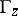consisting of a finite number of Jordan arcs let there be given a single-valued analytic function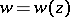which satisfies the following conditions: the values,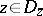, form part of the domainwith boundaryconsisting of a finite number of Jordan arcs; the function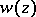can be continuously extended onto some set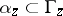consisting of a finite number of arcs; and the values ofonform part of a setwith boundary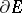consisting of a finite number of Jordan arcs. Under these conditions one has, at any pointat which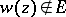,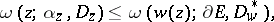(1)
where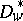denotes the subdomain of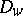such that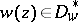and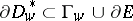. If (1) becomes an equality at any point, then equality will be valid everywhere in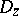. In particular, for a one-to-one conformal mapping fromontoone has the identity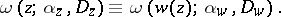The principle of harmonic measure, including its numerous applications , , was established by R. Nevanlinna. In particular, a corollary of the principle is the two-constants theorem, which implies, in turn, that for a functionthat is holomorphic in a domain, the maximum value of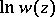on the level lineis a convex function of the parameter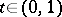.
The principle of harmonic measure has been generalized to holomorphic functions,, of several complex variables,.